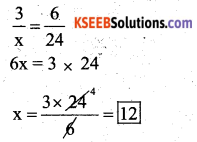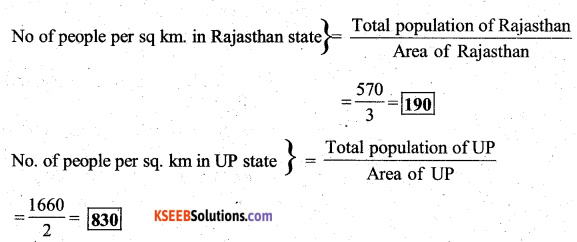# KSEEB Solutions for Class 7 Maths Chapter 8 Comparing Quantities Ex 8.1

Students can Download Chapter 8 Comparing Quantities Ex 8.1, Question and Answers, Notes Pdf, KSEEB Solutions for Class 7 Maths, Karnataka State Board Solutions help you to revise complete Syllabus and score more marks in your examinations.

## Karnataka State Syllabus Class 7 Maths Chapter 8 Comparing Quantities Ex 8.1

Question 1.
Find the ratio of :
a) 5 to 5o paise
Solution:
Rs. 5 : 50 paise
5 × 100 : 50 paise
500 paise : 50 paise (Dividing by 50)
10 : 1

b) 15 kg to 210 g
15 kg: 210 g
15 × 1000: 210 g
15000 g : 210 g (Dividing by 30) 500 : 7

c) 9 m to 27 cm
9 m : 27 cm
9 × 100 : 27 cm
900 cm : 27 cm (Dividing by 9)
100 : 3d) 30 days to 36 hours
30 days : 36 hours
30 × 24 : 36 hours
720 hours : 36 hours (Dividing by 36)
20 : 1

Question 2.
In a computer lab, there are 3 computers for every 6 students. How many computers will be needed for 24 students?
Solution:
Let ‘x’ computers to be needed for 24 students. Then the ratio will be 3 : x = 6 : 24∴ 24 students need 12 computers.

Question 3.
Population of Rajasthan = 570 lakhs and population of UP = 1660 lakhs. Area of Rajasthan = 3 lakh km2 and area of UP= 2 lakh km2.
Solution:
Population of Rajasthan = 570 lakhs
Population of UP = 1660 lakhs
Area of Rajasthan = 3 lakh sq. kms
Area of U.P = 2 lakh sq. kms

i) How many people are there per km2 in both these States ?
Solution:ii) Which State is less populated?
Solution:
Rajasthan State is less populated.